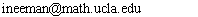Math 223S, Topics in Set Theory, Forcing. Spring 2011

Time and Place: MWF 11-11:50am, in MS 5148.

Instructor: Itay Neeman.
Office: MS 6334.
Email:Phone: 794-5317.
Office hours: Mondays and Wednesdays, 12-1pm.

The class will cover forcing, a technique introduced by Cohen to prove the independence of the Continuum Hypothesis from the axioms of mathematics.

Recall that two sets $A$ and $B$ have the same cardinality if there is a bijective function $f \colon A\rightarrow B$. The Continuum Hypothesis states that every infinite subset of ${\mathbb R}$ has either the same cardinality as ${\mathbb R}$, or the same cardinality as ${\mathbb N}$; there are no cardinalities in between.

It turns out that the Continuum Hypothesis is {\em independent}, meaning neither provable nor refutable, from the axioms of mathematics. That it cannot be refuted was shown by G\"odel (1940's) and that it cannot be proved was shown by Cohen (1960's). Cohen's technique has since been used in proofs of many other independence results. For example Solovay used it to show that the existence of a non-measurable set of reals cannot be proved without the axiom of choice.

The class will cover forcings from the basics, but will progress very quickly to more advanced applications. For the first three weeks we will follow Palumbo's lecture notes on forcing from the UCLA Logic Center 2009 Summer School. (We will go through them very quickly, and students are advised to read ahead of time.) We will then continue with the sections on iterated forcing in Kunen's book, and finally move to the subject of proper forcing, among the most central in the modern study of forcing, largely using Abraham's paper in the Handbook of Set Theory.

Reference Texts:

Grading and assignments: Students will be asked to solve questions from a list assigned to the class, and present the solutions in class. Grading will be based on the presentations. The list of assigned questions (updated as the term progresses) is:

• Assignment 1, due Friday 4/8: read days 1, 2, and 3 in Palumbo's lecture notes, and solve the exercises for these days.
• Assignment 2, due Friday 4/22: read days 7, 8, 9, 10, and 11 in Palumbo's lecture notes, and solve the exercises for these days.
• Assignment 3, due Friday 5/6: read days 4, 5, and 6 in Palumbo's lecture notes, and solve the exercises for these days, and the additional challenging MA problems.
• Assignment 4, due Friday 5/20: from Chapter 7 of Kunen's book, exercises E1 through E5 (on automorphisms and AC), G7, G8 (posets of functions), and H1, H2, H6, H7, H8, H9 (specific forcing constructions).
• Assignment 5, due (and presented) Friday 6/3: from Chapter 8 of Kunen's book, exercises H3 through H6 (on exponentiation of singular cardinals); and from Chapter 2 of Kunen's book, exercises 40 and 41.
You should work on all assigned questions. Let me know on the due date which questions you succeeded in solving. I will ask you to present some of them the following week in class.